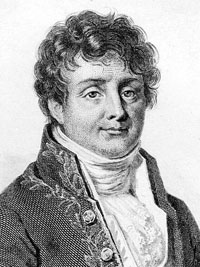Joseph Fourier

1768–1830

French mathematician

The profound study of nature is the most fertile source of mathematical discoveries.

TOPICS: nature, discovery

SOURCE: Morris Kline, Mathematical Thought from Ancient to Modern Times, New York, 1972

The differential equations of the propagation of heat express the most general conditions, and reduce the physical questions to problems of pure analysis, and this is the proper object of theory.\r\n

TOPICS: heat, differential equations, physics, theory

SOURCE: Analytical Theory of Heat

Heat, like gravity, penetrates every substance of the universe, its rays occupy all parts of space. The object of our work is to set forth the mathematical laws which this element obeys. The theory of heat will hereafter form one of the most important branches of general physics.

TOPICS: heat, physics

SOURCE: Analytical Theory of Heat

Mathematical Analysis is as extensive as nature herself.

TOPICS: analysis, nature